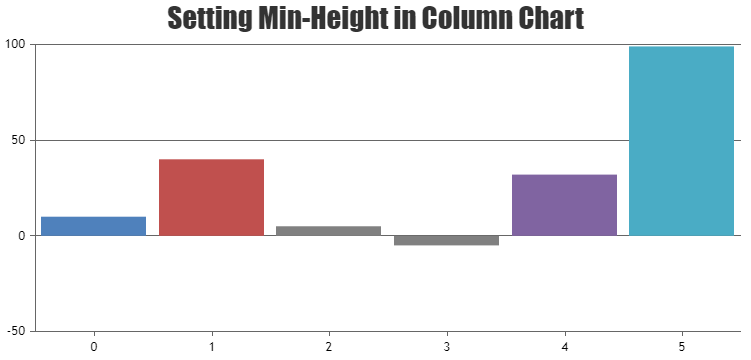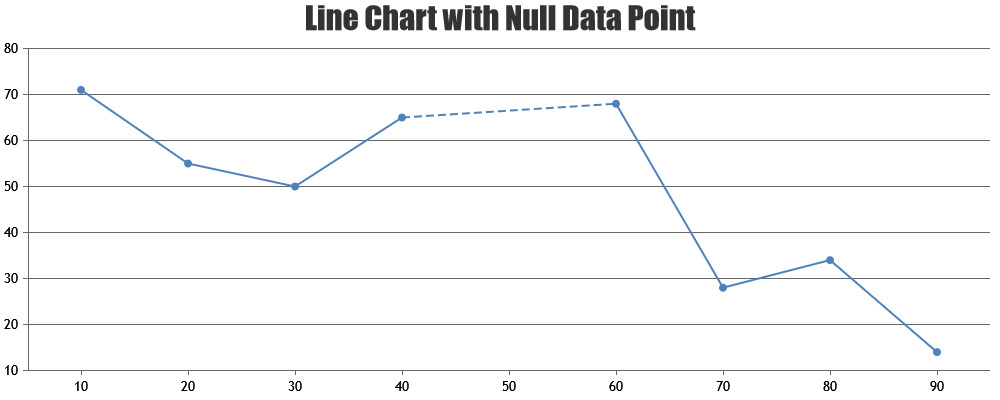Home Forums Chart Support Issue with logarithmic and value 0

# Issue with logarithmic and value 0

Viewing 8 posts - 1 through 8 (of 8 total)
• #35917

Here is a simple example: https://jsfiddle.net/Latbc82r/. As you see if I set logarithmic to true on axisY, then if the value is 0, the column will be shown full height

#35928

A logarithmic axis can only plot positive values. There simply is no way to put negative values or zero on a logarithmic axis.

Fundamental: If 10L = Z, then L is the logarithm (base 10) of Z. If L is a negative value, then Z is a positive fraction less than 1.0. If L is zero, then Z equals 1.0. If L is greater than 0, then Z is greater than 1.0. Note that there no value of L will result in a value of Z that is zero or negative. Logarithms are simply not defined for zero or negative numbers.

Vishwas R
Team CanvasJS

#35936

I understand the tech limitation. But from user’s perspective, if the value is 0 it is not supposed to show a max height column. Is it possible we handle the value 0 specifically?

#35952

As zero value is not supported in logarithmic axis, you can set the value to null by parsing through the datapoints before rendering. Please find the code-snippet below.

``````function parseZeroValue() {
var data = chart.options.data;
for(var i = 0; i < data.length; i++) {
for(var j =0; j < data[i].dataPoints.length; j++) {
var yValue = data[i].dataPoints[j].y;
if(yValue === 0) {
data[i].dataPoints[j].y = null; //Set this to some value like 0.01, if you like to show column
data[i].dataPoints[j].toolTipContent = ("{x}: " + yValue);
}
}
}
}``````

Please take a look at this JSFiddle for complete code. Also refer to this JSFiddle which shows how to set minimum height in column chart.Vishwas R
Team CanvasJS

#35964

This seems to work for column chart, but fails on spline chart: https://jsfiddle.net/0setgxqf/

#35977

In case of line / spline chart, by default line will be broken when datapoint with null value is present. You can connect the non-null values directly by setting connectNullData property to true – which draws a dashed line to connect non-null datapoints. The type of this line can be changed by setting nullDataLineDashType property. Please refer to the code-snippet below.

``````...
data: [{
...
type: "line",
connectNullData: true,
nullDataLineDashType: "solid",
...
}]
...
``````Please take a look at this updated JSFiddle for complete code.

Vishwas R
Team CanvasJS

#35981

But with this workaround, the value 0 is not respected. In your example, at x=20, the line should go down to 0 then go back up, but it just bypassed it

#36000

As mentioned in previous reply, 0 is not supported in logarithmic axis. However, as addressed in previously shared JSFiddle (line no 29), you can either set it to null or to some value like 0.1, 0.00001, etc. to keep the continuity in the line. Please take a look at this updated JSFiddle.

Vishwas R
Team CanvasJS

Viewing 8 posts - 1 through 8 (of 8 total)

You must be logged in to reply to this topic.